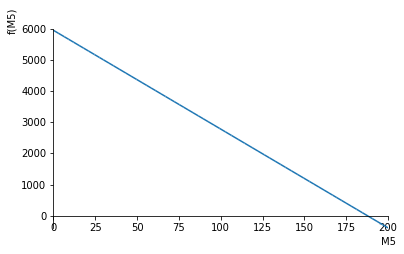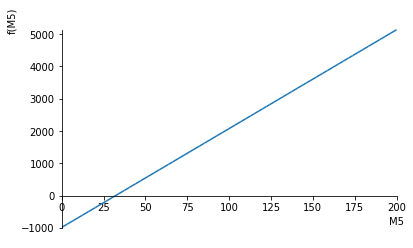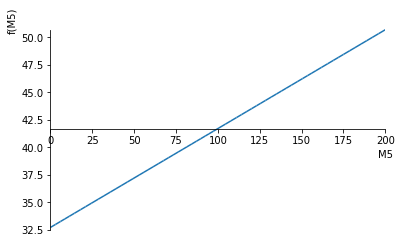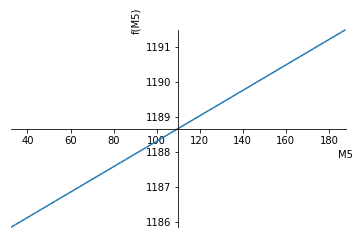This notebook contains course material from CBE20255 by Jeffrey Kantor (jeff at nd.edu); the content is available on Github. The text is released under the CC-BY-NC-ND-4.0 license, and code is released under the MIT license.

# Separating Milk¶

## Summary¶

This example describes a situation where the material balances and problem specifications do not result in a unique solution. Instead, there is at least one degree of freedom that must be resolved by further considerations. In this case, those considerations include the testing for physically meaningful answers, and to maximize a business objective. What you should learn from this example -

• Degrees of freedom may remain after all of the material balance and process specifications have are satisfied.
• The importance of testing for feasible solutions.
• How to use a degree of freedom to maximize a process objective.

## Problem Statement¶

A dairy processor can separate raw milk into a number of commodity products, each with a price determined by the spot market. An example of commodity prices and product specifications is given in this table.A commercial milk separator manufactured by Gruppo Pieralsi.

Component Fat (wt%) Non-fat Solids (wt%) Price
Raw Milk 3.85 8.85
Regular Milk 3.25 8.25 21.48 \$/cwt Skim Milk 0.10 8.25 16.01 \$/cwt
Nonfat Dry Milk 0.0 100.0 1.95 \$/lb Butterfat 100 0.0 1.77 \$/lb

Suppose a source can provide 5000 lb/hour of raw whole milk containing 3.85 wt% fat, and 8.85 wt% non-fat solids. The processor can produce a slate of products consisting of regular milk, skim milk, non-fat dry milk, and butterfat in whatever quantities are needed. Specify the product flows that maximize the revenue returned by this operation. What is the highest price the processor can pay for raw milk and still generate a positive cash flow?

(See The Daily Dairy Report for more background on the diary industry.)

## Solution¶

In :
%matplotlib inline
import sympy as sym
sym.init_printing()


### Define Streams¶

The first step is to label the streams, and create mass flow variables corresponding to each stream.

In :
sym.var(['M1','M2','M3','M4','M5'])

Out:
$$\left [ M_{1}, \quad M_{2}, \quad M_{3}, \quad M_{4}, \quad M_{5}\right ]$$
In :
prods = {};
prods[M1] = "Raw Milk"
prods[M2] = "Regular Milk Product"
prods[M3] = "Skim Milk Product"
prods[M4] = "Nonfat Dry Milk Product"
prods[M5] = "Butterfat Product"
prods

Out:
{M1: 'Raw Milk',
M2: 'Regular Milk Product',
M3: 'Skim Milk Product',
M4: 'Nonfat Dry Milk Product',
M5: 'Butterfat Product'}

### Material Balances¶

In :
mbal = [
sym.Eq(M1, M2 + M3 + M4 + M5),
sym.Eq(0.0385*M1, 0.0325*M2 + 0.001*M3 + 0*M4 + 1.00*M5),
sym.Eq(0.0885*M1, 0.0825*M2 + 0.0825*M3 + 1.00*M4 + 0*M5)
]

for m in mbal:
print(m)

Eq(M1, M2 + M3 + M4 + M5)
Eq(0.0385*M1, 0.0325*M2 + 0.001*M3 + 1.0*M5)
Eq(0.0885*M1, 0.0825*M2 + 0.0825*M3 + 1.0*M4)


### Specifications¶

In :
specs = [
sym.Eq(M1, 5000)
]


### Finding Feasible Solutions to the Material Balances¶

In :
soln = sym.solve(specs + mbal)

for m in soln.keys():
print(m, ":", soln[m])

M4 : 0.0899182561307902*M5 + 32.6975476839237
M3 : 30.6215129103412*M5 - 986.116517451667
M2 : -31.711431166472*M5 + 5953.41896976774
M1 : 5000.00000000000


Let's repeat this step, this time specifying precisely which variables to solve for. The solutions will be found in terms of the remaining variables of the problem.

In :
soln = sym.solve(specs + mbal,[M1,M2,M3,M4])

for m in soln.keys():
print(m, ":", soln[m])

M1 : 5000.00000000000
M2 : -31.711431166472*M5 + 5953.41896976774
M3 : 30.6215129103412*M5 - 986.116517451667
M4 : 0.0899182561307902*M5 + 32.6975476839237


What are the feasible values of $M_5$?

In :
sym.plot(M2.subs(soln),(M5,0,200))
print(sym.solve(M2.subs(soln),M5))[187.737315875614]

In :
sym.plot(M3.subs(soln),(M5,0,200))
print(sym.solve(M3.subs(soln),M5))[32.2033898305085]

In :
sym.plot(M4.subs(soln),(M5,0,200))
print(sym.solve(M4.subs(soln),M5))[-363.636363636364]

In :
M5_max = sym.solve(M2.subs(soln))
M5_min = sym.solve(M3.subs(soln))


### Finding a Solution to Maximize Revenue¶

In :
Revenue = 21.48*M2.subs(soln)/100.0 \
+ 16.01*M3.subs(soln)/100.0 \
+ 1.95*M4.subs(soln) \
+ 1.77*M5

print(Revenue)

0.0362294018424814*M5 + 1184.67735824575

In :
sym.plot(Revenue,(M5,M5_min,M5_max))Out:
<sympy.plotting.plot.Plot at 0x1162a8668>
In :
opt = [sym.Eq(M5_min,M5)]

soln = sym.solve(mbal + specs + opt)

for m in soln.keys():
print("{0:2s} : {1:8.1f} lb/hr  {2}".format(str(m), soln[m], str(prods[m])))

M1 :   5000.0 lb/hr  Raw Milk
M2 :   4932.2 lb/hr  Regular Milk Product
M3 :      0.0 lb/hr  Skim Milk Product
M4 :     35.6 lb/hr  Nonfat Dry Milk Product
M5 :     32.2 lb/hr  Butterfat Product


Revenue and Milk Price Calculations

In :
print("   Maximum Revenue = ${0:8.2f}/hr".format(Revenue.subs(soln))) print("Max Raw Milk Price =${0:8.2f}/lb".format(Revenue.subs(soln)/5000.0))

   Maximum Revenue = $1185.84/hr Max Raw Milk Price =$    0.24/lb

In [ ]: